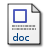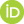# On Tins and Tin-openers

Liston, Michael (2009) On Tins and Tin-openers. In: UNSPECIFIED.Microsoft Word (.doc) On_Tins_and_Tin-openers.doc Download (75kB)

## Abstract

Most science requires applied mathematics. This truism underlies the Quine-Putnam indispensability argument: we cannot be mathematical nominalists without rejecting whole swaths of good science that are seamlessly linked with mathematics. One style of response (e.g. Field’s program) accepts the challenge head-on and attempts to show how to do science without mathematics. There is some consensus that the response fails because the nominalistic apparatus deployed either is not extendible to all of mathematical physics or is merely a deft reconstrual equivalent to standard mathematics. A second style of response (suggested, e.g., by Balaguer and Maddy) denies that indispensability entails realism: when we mathematize a physical problem we treat its physical content as if it were the mathematical representation; provided the two are sufficiently similar, we can use the mathematics to draw conclusions about the physics; even if we cannot represent physical facts without mathematical tools, as-if-fictionalism is reasonable. In this paper I argue that uses of mathematics in science reach deeper than is appreciated by this second response and, indeed, in the more general literature. More specifically, our confidence that we can use the mathematics to draw conclusions about the physics itself depends on mathematics. If the mathematical premises we employ in concluding that a certain application is trustworthy are false, we may lack a justification for supposing that the application will reliably lead us from correct input to correct output. For example, solutions to many physical problems require the determination of a function satisfying a differential equation. Sometimes (e.g., if the differential equation is linear) the existence of a solution for initial value problems can be established directly; where direct methods fail, the existence of a solution must be established indirectly, generally by constructing a sequence of functions that converges to a limit function that satisfies the initial value problem. Moreover, the solution often cannot be evaluated by analytic methods, and scientists must rely on finite element numerical methods to approximate the solution. Mathematical analysis of errors provides further useful information governing the choice of approximation method and of the step size and number of elements needed for the approximation to reach a desired precision. Mathematical physicists rely on the background mathematical theories (e.g., theory of differential equations) presupposed in proving the existence of the solutions and approximating them. It is difficult to see how they could do this while adding the fictionalist disclaimer, “But, you know, I don’t believe any of the mathematics I’m using”. It is difficult to see how a fictionalist pursuing the second strategy can account for the soundness of mathematical reasoning in mathematical physics and elsewhere in the sciences. The paper will fill out this argument by appeal to examples and attempt to make clear (a) how mathematics is indispensable to understanding – and thus underwriting our confidence in – applications that would otherwise be shaky approximations and idealizations and (b) how this role is difficult to square with fictionalism.

 Export/Citation: EndNote | BibTeX | Dublin Core | ASCII/Text Citation (Chicago) | HTML Citation | OpenURL
 Social Networking:

Item Type: Conference or Workshop Item (UNSPECIFIED)
Creators:
CreatorsEmailORCIDListon, Michael
Keywords: Indispensability arguments, mathematical fictionalism, arealism, nominalism, philosophy of mathematics
Subjects: Specific Sciences > Mathematics
Depositing User: michael liston
Date Deposited: 01 Apr 2010
Item ID: 5249
Subjects: Specific Sciences > Mathematics
Date: 2009
URI: http://philsci-archive.pitt.edu/id/eprint/5249

### Monthly Views for the past 3 yearsView Item